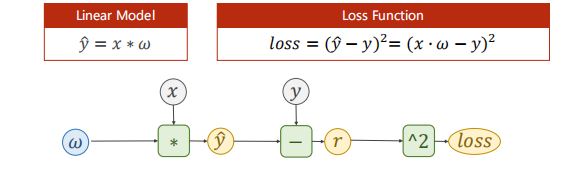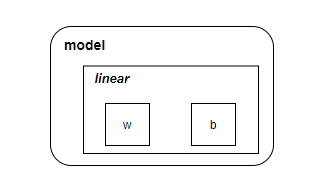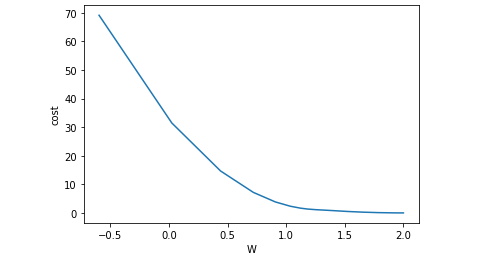## 回顾

之前我们用纯python实现了一个线性模型。现在，以第一篇文章的例子来，试着用 Pytorch 提供的工具来实现一个线性模型。## Pytorch 写神经网络的套路

• 准备数据集
• 设计模型类（用来计算 $\hat{y}$）
• 构造损失函数和优化器（optmizer）
• 训练模型：
• 前馈计算Loss
• 反向传播Loss （梯度下降算法）
• 更新

## 开始

1.准备数据集：

在pytorch中，采用的是小批量地图下降算法，因此，$X$ 和 $\hat{Y}$ 是一个多维的 Tensor. 展开后的模型为： $$\begin{bmatrix} \hat{y}_1 \\ \hat{y}_2 \\ \hat{y}_3 \\ \dots \\ \end{bmatrix} = w \cdot \begin{bmatrix} x_1 \\ x_2 \\ x_3 \\ \dots \\ \end{bmatrix} + b$$ 上式的" $\cdot$ " 表示的是数乘，要与矩阵乘法 " $\times$ " 区分开来。$w \cdot \begin{bmatrix} x_1 \\ x_2 \\ x_3 \\ \dots \\ \end{bmatrix}$会触发广播机制: $$w \Rightarrow \begin{bmatrix} w \\ w \\ w \\ \dots \\ \end{bmatrix}$$

2. 定义模型类：

模型类中 init 函数与 forward 函数时必须实现的。反而 backward 函数无需定义，因为torch会根据计算图自动计算梯度。但是如果你认为默认的梯度计算性能不佳可以自己对torch内的Function类中实现的反向传播功能进行重载。

model 对象大致结构如下：3. 构造损失函数和优化器：

当我们计算得到预测值 $\begin{bmatrix} \hat{y}_1 \ \hat{y}_2 \ \hat{y}_3 \ \dots \ \end{bmatrix}$ 后, 根据$Loss = (\hat{Y}- Y)^2$得损失为：

Pytorch中其他的优化器：

4. 模型训练：

5. 打印查看收敛过程：